# Test: The Laplace Transform

## 20 Questions MCQ Test GATE Electrical Engineering (EE) 2023 Mock Test Series | Test: The Laplace Transform

Description
Attempt Test: The Laplace Transform | 20 questions in 60 minutes | Mock test for Railways preparation | Free important questions MCQ to study GATE Electrical Engineering (EE) 2023 Mock Test Series for Railways Exam | Download free PDF with solutions
QUESTION: 1

### Given the transform pair below. Determine the time signal y(t) and choose correct option.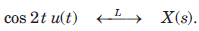​ ​ ​ Que: Y(s) = (s + 1)X(s)

Solution: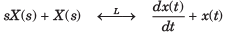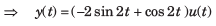QUESTION: 2

### Given the transform pair below. Determine the time signal y(t) and choose correct option.Que: Y(s) = X(3s)

Solution: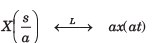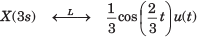QUESTION: 3

### Given the transform pair below. Determine the time signal y(t) and choose correct option.Que: Y(s) = X(s + 2)

Solution: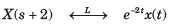QUESTION: 4

Unilateral Laplace Transform is applicable for the determination of linear constant coefficient differential equations with ________

Solution:
QUESTION: 5

Given the transform pair below. Determine the time signal y(t) and choose correct option.Que: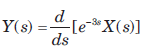Solution: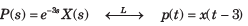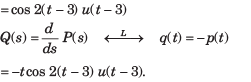QUESTION: 6

Given the transform pair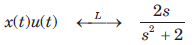Determine the Laplace transform Y(s) of the given time signal in question and choose correct option.

Que: y(t) = x(t - 2)

Solution: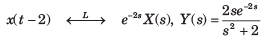QUESTION: 7

Given the transform pairDetermine the Laplace transform Y(s) of the given time signal in question and choose correct option.

Que: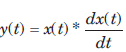Solution: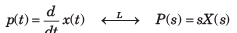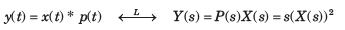QUESTION: 8

Given the transform pairDetermine the Laplace transform Y(s) of the given time signal in question and choose correct option.

Que: y(t) = e-t x(t)

Solution: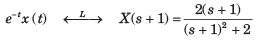QUESTION: 9

Given the transform pairDetermine the Laplace transform Y(s) of the given time signal in question and choose correct option.

Que: y(t) = 2tx(t)

Solution: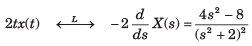QUESTION: 10

Determine the bilateral laplace transform and choose correct option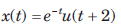​ ​ ​

Solution: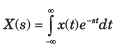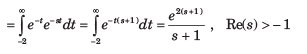QUESTION: 11

Determine the bilateral laplace transform and choose correct option.

x(t) = u(-t + 3)

Solution: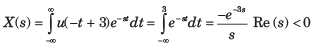QUESTION: 12

Determine the bilateral laplace transform and choose correct option

y(t) = δ(t + 1)

Solution: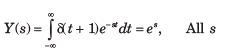QUESTION: 13

Determine the bilateral laplace transform and choose correct option

x(t) = sin t u(t)

Solution: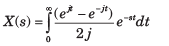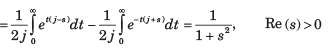QUESTION: 14

Determine the bilateral laplace transform and choose correct option.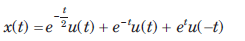Solution: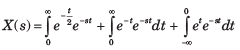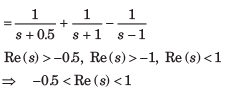QUESTION: 15

Determine the bilateral laplace transform and choose correct option.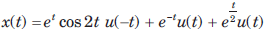Solution: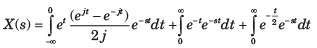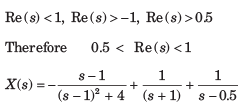QUESTION: 16

Determine the bilateral laplace transform and choose correct option.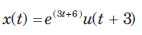​ ​ ​

Solution: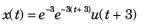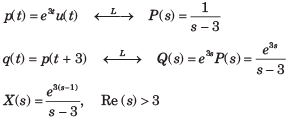QUESTION: 17

Where is the ROC defined or specified for the signals containing causal as well as anti-causal terms?

Solution:
QUESTION: 18

The transform function H(s) of a causal system is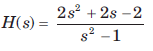The impulse response is

Solution:

For a causal system h(t) = 0 for t < 0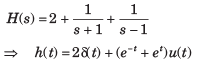QUESTION: 19

The transfer function H(s) of a stable system is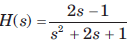The impulse response is

Solution: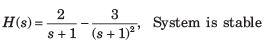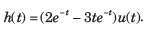QUESTION: 20

The transfer function H(s) of a stable system is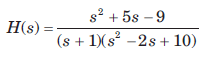The impulse response is

Solution: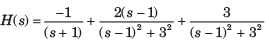System is stable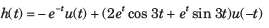Use Code STAYHOME200 and get INR 200 additional OFF Use Coupon Code